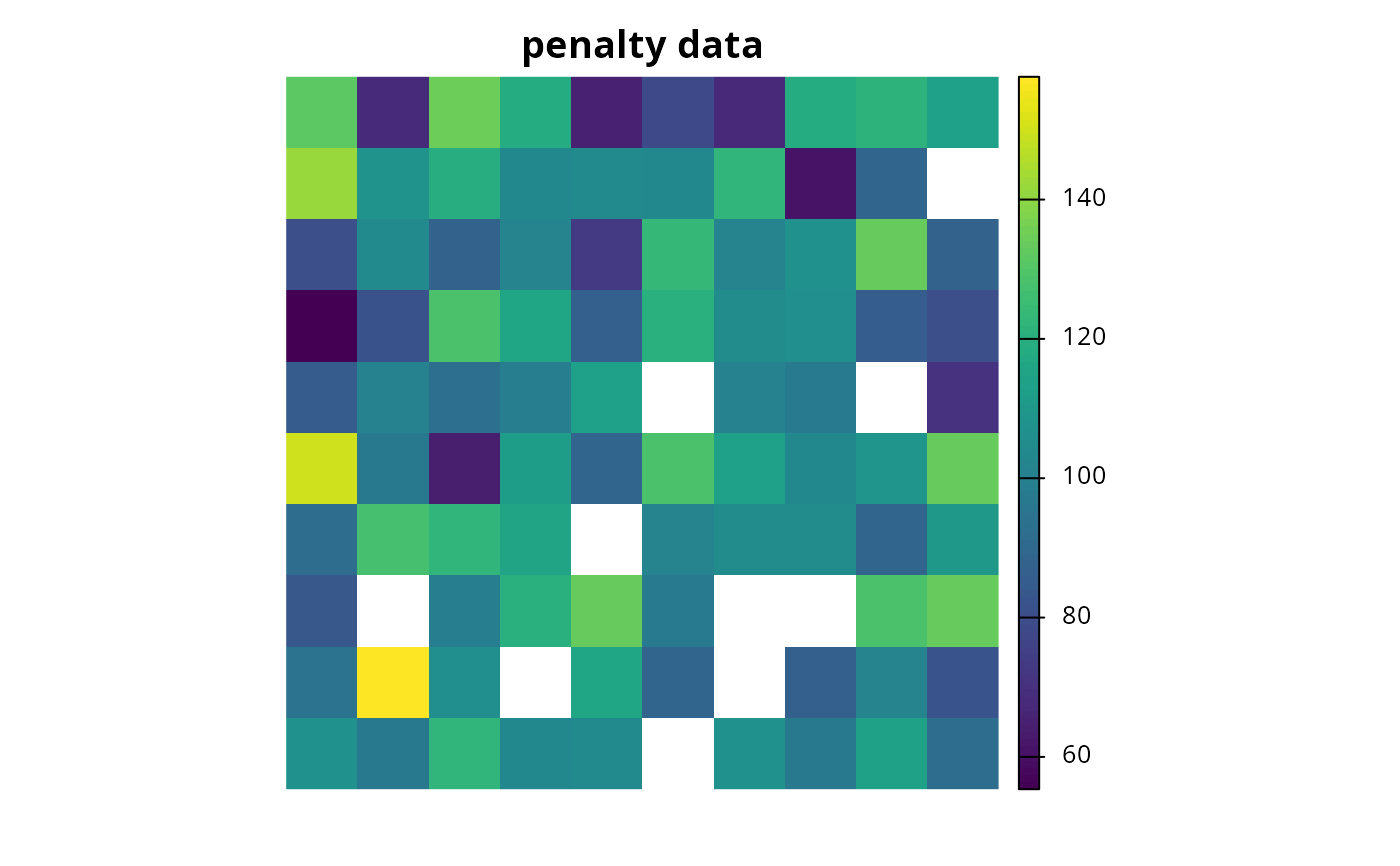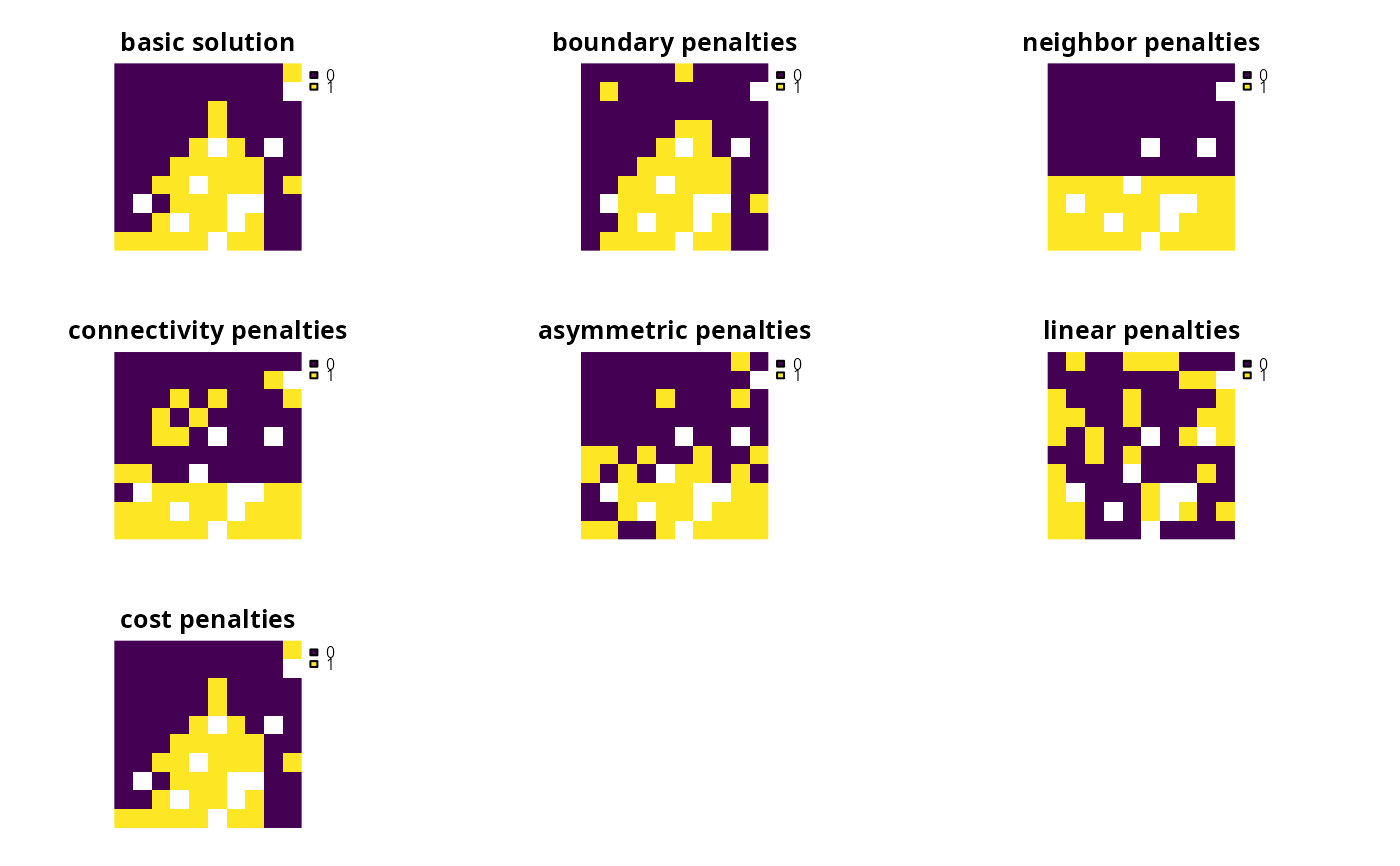A penalty can be applied to a conservation planning problem to penalize solutions according to a specific metric. They directly trade-off with the primary objective of a problem (e.g., the primary objective when using add_min_set_objective() is to minimize solution cost).

## Details

Both penalties and constraints can be used to modify a problem and identify solutions that exhibit specific characteristics. Constraints work by invalidating solutions that do not exhibit specific characteristics. On the other hand, penalties work by specifying trade-offs against the primary problem objective and are mediated by a penalty factor.

The following penalties can be added to a conservation planning problem():

add_boundary_penalties()

Add penalties to a conservation problem to favor solutions that have planning units clumped together into contiguous areas.

add_asym_connectivity_penalties()

Add penalties to a conservation problem to account for asymmetric connectivity.

add_connectivity_penalties()

Add penalties to a conservation problem to account for symmetric connectivity.

add_linear_penalties()

Add penalties to a conservation problem to favor solutions that avoid selecting planning units based on a certain variable (e.g., anthropogenic pressure).

Other overviews: constraints, decisions, importance, objectives, portfolios, solvers, summaries, targets

## Examples

# \dontrun{
sim_pu_raster <- get_sim_pu_raster()
sim_features <- get_sim_features()

# create basic problem
p1 <-
problem(sim_pu_raster, sim_features) %>%

# create problem with boundary penalties
p2 <- p1 %>% add_boundary_penalties(5, 1)

# create connectivity matrix based on spatial proximity
scm <- terra::as.data.frame(sim_pu_raster, xy = TRUE, na.rm = FALSE)
scm <- 1 / (as.matrix(dist(as.matrix(scm))) + 1)

# remove weak and moderate connections between planning units to reduce
# run time
scm[scm < 0.85] <- 0

# create problem with connectivity penalties
p3 <- p1 %>% add_connectivity_penalties(25, data = scm)

# create asymmetric connectivity data by randomly simulating values
acm <- matrix(runif(ncell(sim_pu_raster) ^ 2), ncol = ncell(sim_pu_raster))
acm[acm < 0.85] <- 0

# create problem with asymmetric connectivity penalties
p4 <- p1 %>% add_asym_connectivity_penalties(1, data = acm)

# create problem with linear penalties,
# here the penalties will be based on random numbers to keep it simple

# simulate penalty data
sim_penalty_raster <- simulate_cost(sim_pu_raster)

# plot penalty data
plot(sim_penalty_raster, main = "penalty data", axes = FALSE)# create problem with linear penalties, with a penalty scaling factor of 100
p5 <- p1 %>% add_linear_penalties(100, data = sim_penalty_raster)

# solve problems
s <- c(solve(p1), solve(p2), solve(p3), solve(p4), solve(p5))
names(s) <- c(
"basic solution", "boundary penalties", "connectivity penalties",
"asymmetric penalties", "linear penalties"
)

# plot solutions
plot(s, axes = FALSE)# }